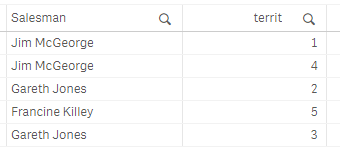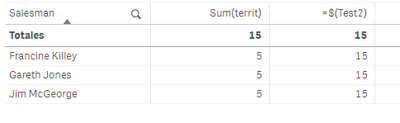# App Development

Announcements
Don't miss the upcoming Q&A with Qlik session on Qlik Application Automation on November 16th! REGISTER NOW
cancel
Showing results for
Did you mean:Contributor III

## Expression in variable not the same formula

Hi all,

I have some data like:My problem is that if I introduce my expresion directly in the table, it works well, but if I introduce the expression in a variable and use it as a measure in the table the result is not the same, it shows as total:Test2=sum(territ)

Labels (1)
• ### qlik sense variable expression total measure

1 Solution

Accepted SolutionsMaster II

If you use =\$(Variable) on a variable containing an equal sign such as =sum(territ), it will be evaluated first, outside the context of the object / row, and the result will be used in your formula.

You could try replacing your variable with Sum(territ) instead of =Sum(territ) and then =\$(Test2) should evaluate within the context of the row. In the example here, I've got =Sum(id) as Test1 and Sum(id) as Test2Master II

If you use =\$(Variable) on a variable containing an equal sign such as =sum(territ), it will be evaluated first, outside the context of the object / row, and the result will be used in your formula.

You could try replacing your variable with Sum(territ) instead of =Sum(territ) and then =\$(Test2) should evaluate within the context of the row. In the example here, I've got =Sum(id) as Test1 and Sum(id) as Test2Tags
Community Browser